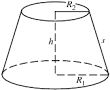## How to Calculate and Solve for the Height and Volume of a Conical Frustum | The Calculator EncyclopediaThe image above is a conical frustum.

To compute the volume of a conical frustum, three essential parameters are needed and this parameters are radius of the lower base (R), radius of the upper base (r) and height (h).

The formula for calculating the volume of a conical frustum:

V = πh(R² + Rr + r²)3

Where;
V = Volume of the conical frustum
R = Radius of the lower base
r = Radius of the upper base
h = Height of the conical frustum

Let’s solve an example;
Find the volume of the conical frustum when the lower base is 5 cm with an upper base of 9 cm and a height of 11 cm.

This implies that;
R = Radius of the lower base = 5 cm
r = Radius of the upper base = 9 cm
h = Height of the conical frustum = 11 cm

V = π(11)((5)² + (5)(9) + (9)²)3
V = (34.557)((25) + (45) + (81))3
V = (34.557)(151)3
V = 5218.1853
V = 1739.39

Therefore, the volume of the conical frustum is 1739.39 cm3.